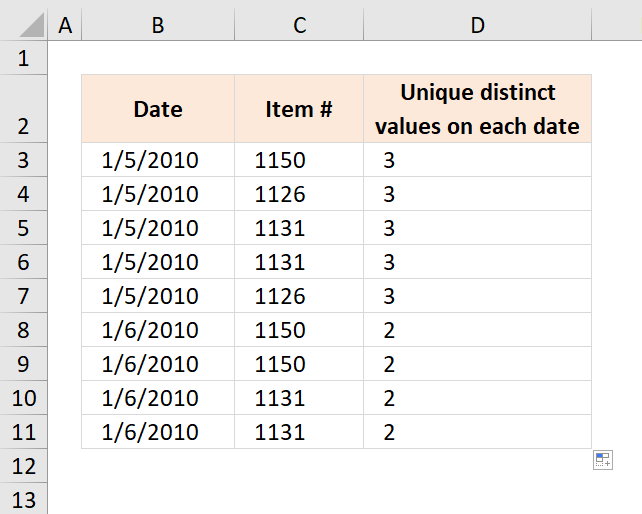Author: Oscar Cronquist Article last updated on January 31, 2019The array formula in cell D3 calculates the number of unique distinct items based on the given date in column B. Unique distinct values are all values but duplicates are merged into one value.

Example, there are five items on date 1/5/2010 in the table above. 1150, 1126, 1131, 1131 and 1126, however there are only three unique distinct items 1150, 1126 and 1131 and that number is what the formula returned in cell D3.

Array formula in D3:

=SUM(--(FREQUENCY(IF(B3=\$B\$3:\$B\$11, COUNTIF(\$C\$3:\$C\$11, "<"&\$C\$3:\$C\$11), ""), (COUNTIF(\$C\$3:\$C\$11, "<"&\$C\$3:\$C\$11)))>0))

To enter an array formula, type the formula in a cell then press and hold CTRL + SHIFT simultaneously, now press Enter once. Release all keys.

The formula bar now shows the formula with a beginning and ending curly bracket telling you that you entered the formula successfully. Don't enter the curly brackets yourself.

Copy cell D3 and paste it down as far as needed.

### Explaining formula in cell D3

#### Step 1 - Calculate alphabetical rank

The COUNTIF function counts values based on a condition or criteria, in this case I use the ampersand to concatenate a less than sign. This will return a number representing the rank in an alphabetically sorted list.

COUNTIF(\$C\$3:\$C\$11, "<"&\$C\$3:\$C\$11)

becomes

COUNTIF({1150;1126;1131;1131;1126;1150;1150;1131;1131},{"<1150";"<1126";"<1131";"<1131";"<1126";"<1150";"<1150";"<1131";"<1131"})

and returns

{6;0;2;2;0;6;6;2;2}

#### Step 2 - Extract numbers based on date

The IF function has three arguments, the first one must be a logical expression. If the expression evaluates to TRUE then one thing happens (argument 2) and if FALSE another thing happens (argument 3).

IF(B3=\$B\$3:\$B\$11, COUNTIF(\$C\$3:\$C\$11, "<"&\$C\$3:\$C\$11), "")

becomes

IF(40183={40183; 40183; 40183; 40183; 40183; 40184; 40184; 40184; 40184},COUNTIF(\$C\$3:\$C\$11,"<"&\$C\$3:\$C\$11),"")

becomes

IF({TRUE; TRUE; TRUE; TRUE; TRUE; FALSE; FALSE; FALSE; FALSE},COUNTIF(\$C\$3:\$C\$11,"<"&\$C\$3:\$C\$11),"")

and returns

{6;0;2;2;0;"";"";"";""}.

#### Step 3 - Cacluate frequency

The FREQUENCY function calculates how often values occur in a range.

FREQUENCY(IF(B3=\$B\$3:\$B\$11, COUNTIF(\$C\$3:\$C\$11, "<"&\$C\$3:\$C\$11), ""), (COUNTIF(\$C\$3:\$C\$11, "<"&\$C\$3:\$C\$11))

becomes

FREQUENCY({6; 0; 2; 2; 0; ""; ""; ""; ""},{6; 0; 2; 2; 0; 6; 6; 2; 2})

and returns

{1;2;2;0;0;0;0;0;0;0}

#### Step 4 - Is number larger than 0 (zero)?

The value is unique distinct if the corresponding number in the array is larger than 0 (zero).

FREQUENCY(IF(B3=\$B\$3:\$B\$11, COUNTIF(\$C\$3:\$C\$11, "<"&\$C\$3:\$C\$11), ""), (COUNTIF(\$C\$3:\$C\$11, "<"&\$C\$3:\$C\$11))>0

becomes

{1;2;2;0;0;0;0;0;0;0}>0

and returns

{TRUE; TRUE; TRUE; FALSE; FALSE; FALSE; FALSE; FALSE; FALSE; FALSE}

#### Step 5 - Convert boolean values

The SUM function can't sum boolean value so we must convert them into their numerical equivalents.

--(FREQUENCY(IF(B3=\$B\$3:\$B\$11, COUNTIF(\$C\$3:\$C\$11, "<"&\$C\$3:\$C\$11), ""), (COUNTIF(\$C\$3:\$C\$11, "<"&\$C\$3:\$C\$11)))>0)

becomes

--({TRUE; TRUE; TRUE; FALSE; FALSE; FALSE; FALSE; FALSE; FALSE; FALSE})

and returns

{1;1;1;0;0;0;0;0;0;0}

#### Step 6 - Sum numbers

The SUM function adds numbers in the array and returns a total.

SUM(--(FREQUENCY(IF(B3=\$B\$3:\$B\$11, COUNTIF(\$C\$3:\$C\$11, "<"&\$C\$3:\$C\$11), ""), (COUNTIF(\$C\$3:\$C\$11, "<"&\$C\$3:\$C\$11)))>0))

becomes

SUM({1;1;1;0;0;0;0;0;0;0})

and returns 3.

### Get the Excel fileCount-unique-occurence-from-each-date.xlsx